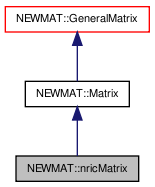# NEWMAT::nricMatrix Class Reference

`#include <newmat.h>`

Inheritance diagram for NEWMAT::nricMatrix:[legend]

## Detailed Description

Definition at line 631 of file newmat.h.

List of all members.

## Public Member Functions

nricMatrix ()
nricMatrix (int m, int n)
nricMatrix (const BaseMatrix &bm)
nricMatrixoperator= (const BaseMatrix &bm)
nricMatrixoperator= (Real f)
nricMatrixoperator= (const nricMatrix &m)
void operator<< (const BaseMatrix &X)
nricMatrix (const nricMatrix &gm)
void ReSize (int m, int n)
void ReSize (const GeneralMatrix &A)
~nricMatrix ()
Real ** nric () const
void CleanUp ()
void MiniCleanUp ()
void operator+= (const Matrix &M)
void operator-= (const Matrix &M)
void operator+= (Real f)
void operator-= (Real f)
void swap (nricMatrix &gm)

## Private Member Functions

GeneralMatrixImage () const
void MakeRowPointer ()
void DeleteRowPointer ()

## Private Attributes

Real ** row_pointer

## Constructor & Destructor Documentation

 NEWMAT::nricMatrix::nricMatrix ( )

Definition at line 639 of file newmat.h.

Referenced by Image().

 NEWMAT::nricMatrix::nricMatrix ( int m, int n )

Definition at line 640 of file newmat.h.

 NEWMAT::nricMatrix::nricMatrix ( const BaseMatrix & bm )

Definition at line 642 of file newmat.h.

 NEWMAT::nricMatrix::nricMatrix ( const nricMatrix & gm )

Definition at line 651 of file newmat.h.

 NEWMAT::nricMatrix::~nricMatrix ( )

Definition at line 655 of file newmat.h.

## Member Function Documentation

 void NEWMAT::nricMatrix::CleanUp ( ) ` [virtual]`

Reimplemented from NEWMAT::GeneralMatrix.

Definition at line 899 of file newmat4.cpp.

 void NEWMAT::nricMatrix::DeleteRowPointer ( ) ` [private]`

Definition at line 874 of file newmat4.cpp.

Referenced by CleanUp(), MiniCleanUp(), operator<<(), operator=(), ReSize(), and ~nricMatrix().

 GeneralMatrix * NEWMAT::nricMatrix::Image ( ) const` [private, virtual]`

Reimplemented from NEWMAT::Matrix.

Definition at line 837 of file newmat4.cpp.

 void NEWMAT::nricMatrix::MakeRowPointer ( ) ` [private]`

Definition at line 862 of file newmat4.cpp.

Referenced by nricMatrix(), operator<<(), operator=(), and ReSize().

 void NEWMAT::nricMatrix::MiniCleanUp ( ) ` [virtual]`

Reimplemented from NEWMAT::GeneralMatrix.

Definition at line 902 of file newmat4.cpp.

 Real** NEWMAT::nricMatrix::nric ( ) const

Definition at line 656 of file newmat.h.

 void NEWMAT::nricMatrix::operator+= ( Real f )

Reimplemented from NEWMAT::Matrix.

Definition at line 661 of file newmat.h.

 void NEWMAT::nricMatrix::operator+= ( const Matrix & M )

Reimplemented from NEWMAT::Matrix.

Definition at line 659 of file newmat.h.

 void NEWMAT::nricMatrix::operator-= ( Real f )

Reimplemented from NEWMAT::Matrix.

Definition at line 662 of file newmat.h.

 void NEWMAT::nricMatrix::operator-= ( const Matrix & M )

Reimplemented from NEWMAT::Matrix.

Definition at line 660 of file newmat.h.

 void NEWMAT::nricMatrix::operator<< ( const BaseMatrix & X )

Reimplemented from NEWMAT::GeneralMatrix.

Definition at line 649 of file newmat.h.

 nricMatrix& NEWMAT::nricMatrix::operator= ( const nricMatrix & m )

Definition at line 647 of file newmat.h.

 nricMatrix& NEWMAT::nricMatrix::operator= ( Real f )

Reimplemented from NEWMAT::Matrix.

Definition at line 646 of file newmat.h.

 nricMatrix& NEWMAT::nricMatrix::operator= ( const BaseMatrix & bm )

Reimplemented from NEWMAT::Matrix.

Definition at line 644 of file newmat.h.

Referenced by operator=().

 void NEWMAT::nricMatrix::ReSize ( const GeneralMatrix & A ) ` [virtual]`

Reimplemented from NEWMAT::Matrix.

Definition at line 311 of file newmat4.cpp.

 void NEWMAT::nricMatrix::ReSize ( int m, int n ) ` [virtual]`

Reimplemented from NEWMAT::Matrix.

Definition at line 652 of file newmat.h.

Referenced by ReSize().

 void NEWMAT::nricMatrix::swap ( nricMatrix & gm )

Definition at line 1051 of file newmat4.cpp.

Referenced by NEWMAT::swap().

## Member Data Documentation

 Real** NEWMAT::nricMatrix::row_pointer` [private]`

Definition at line 635 of file newmat.h.

Referenced by DeleteRowPointer(), MakeRowPointer(), nric(), and swap().

The documentation for this class was generated from the following files:

 newmat11b Generated Mon May 9 04:54:19 2016 by Doxygen 1.6.3